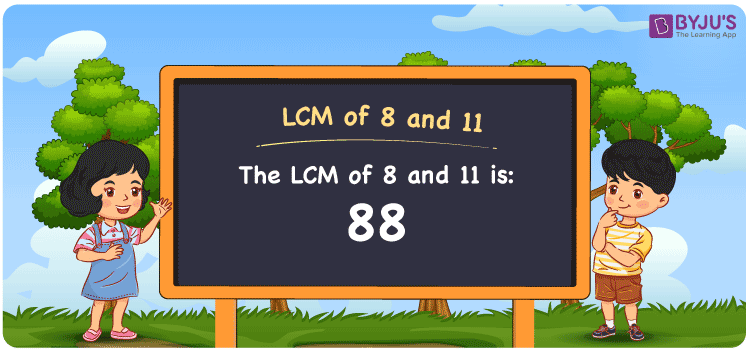Checkout JEE MAINS 2022 Question Paper Analysis : Checkout JEE MAINS 2022 Question Paper Analysis :

# LCM of 8 and 11

LCM of 8 and 11 is 88. Among the multiples of 8 and 11, the value which is evenly divisible gives the LCM. Least common multiple of 8 and 11 is the common multiple obtained using the multiplication operation. (8, 16, 24, 32, 40, ….) and (11, 22, 33, 44, 55, ….) are the multiples of 8 and 11. The LCM of two numbers is determined using methods like the listing of multiples, prime factorization and division.

## What is LCM of 8 and 11?

The answer to this question is 88. The LCM of 8 and 11 using various methods is shown in this article for your reference. The LCM of two non-zero integers, 8 and 11, is the smallest positive integer 88 which is divisible by both 8 and 11 with no remainder.## How to Find LCM of 8 and 11?

LCM of 8 and 11 can be found using three methods:

• Prime Factorisation
• Division method
• Listing the multiples

### LCM of 8 and 11 Using Prime Factorisation Method

The prime factorisation of 8 and 11, respectively, is given by:

8 = 2 x 2 x 2 = 2³

11 = 11¹

LCM (8, 11) = 88

### LCM of 8 and 11 Using Division Method

We’ll divide the numbers (8, 11) by their prime factors to get the LCM of 8 and 11 using the division method (preferably common). The LCM of 8 and 11 is calculated by multiplying these divisors.

 2 8 11 2 4 11 2 2 11 11 1 11 x 1 1

No further division can be done.

Hence, LCM (8, 11) = 88

### LCM of 8 and 11 Using Listing the Multiples

To calculate the LCM of 8 and 11 by listing out the common multiples, list the multiples as shown below:

 Multiples of 8 Multiples of 11 8 11 16 22 24 33 32 44 40 55 48 66 56 77 64 88 72 99 80 – 88 – 96 –

LCM (8, 11) = 88

## Video Lesson on Applications of LCM## LCM of 8 and 11 Solved Examples

Question: Which is the smallest number exactly divisible by 8 and 11?

Solution:

We know that

LCM is the smallest number exactly divisible by 8 and 11.

Multiples of 8 = 8, 16, 24, 32, 40, ….

Multiples of 11 = 11, 22, 33, 44, 55, 66, 77, …..

Hence, the LCM of 8 and 11 is 88.

## Frequently Asked Questions on LCM of 8 and 11

### Which methods can be used to find the LCM of 8 and 11?

The methods which can be used to find the LCM of 8 and 11 are

Prime Factorisation

Division method

Listing the multiples

### With the help of the prime factorisation method, find the LCM of 8 and 11.

We should know the factors first to find the LCM

8 = 2 x 2 x 2 = 2³

11 = 11¹

LCM is the product of prime factors raised to the highest exponent among 8 and 11.

LCM of 8 and 11 = 88

### Find the GCF if the LCM of 8 and 11 is 88.

LCM x GCF = 8 x 11

As the LCM = 88

88 x GCF = 88

GCF = 88/88 = 1